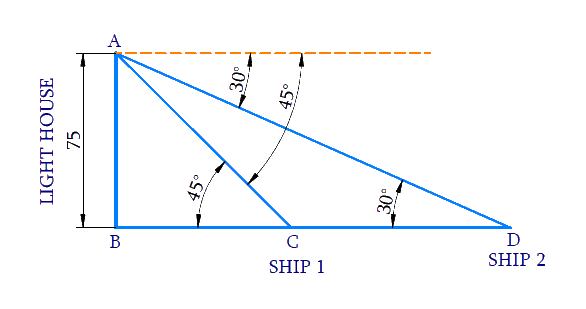# Ex.9.1 Q13 Some Applications of Trigonometry Solution - NCERT Maths Class 10

Go back to  'Ex.9.1'

## Question

As observed from the top of a $$75\,\rm{m}$$ high lighthouse from the sea-level, the angles of depression of two ships are $$30^\circ$$ and $$45^\circ.$$ If one ship is exactly behind the other on the same side of the lighthouse, find the distance between the two ships.

Video Solution
Some Applications Of Trigonometry
Ex 9.1 | Question 13

## Text Solution

What is Known?

(i) Height of the lighthouse $$=75\,\rm{m}$$

(ii) Angles of depression of two ships from the top of the lighthouse are $$30^\circ$$ and $$45^\circ.$$

What is Unknown?

Distance between the two shipsReasoning:

Let the height of the lighthouse from the sea-level is $$AB$$ and the ships are $$C$$ and $$D$$. The angles of depression of the ships $$C$$ and $$D$$ from the top $$A$$ of the lighthouse, are $$45^\circ$$ and $$60^\circ$$ respectively.

Trigonometric ratio involving $$AB, BC, BD$$ and angles is tan$$\theta$$.

Distance between the ships, $$CD = BD − BC$$

Steps:

In $$\Delta ABC$$,

\begin{align} \tan {{45}^{0}}&=\frac{AB}{BC} \\ 1&=\frac{75}{BC} \\ BC&=75\, \end{align}

In $$\Delta ABD$$,

\begin{align} \tan {{30}^{0}}&=\frac{AB}{BD} \\ \frac{1}{\sqrt{3}}&=\frac{75}{BD} \\ BD&=75\sqrt{3} \end{align}

Distance between two ships

$$CD=BD-BC$$

\begin{align}CD&=75\sqrt{3}-75 \\ &=75\left( \sqrt{3}-1 \right) \end{align}

Distance between two ships $$C D = 75 ( \sqrt { 3 } - 1 ) \, \rm{m}$$

Learn from the best math teachers and top your exams

• Live one on one classroom and doubt clearing
• Practice worksheets in and after class for conceptual clarity
• Personalized curriculum to keep up with school